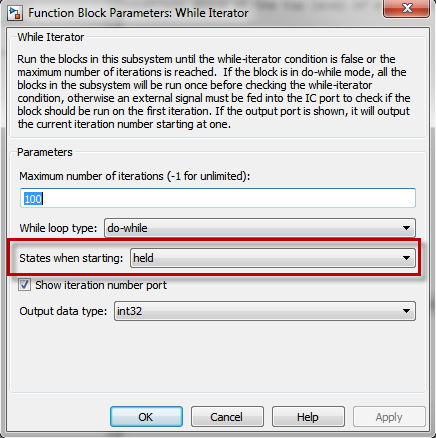# Implementing an iterative algorithm using the While Iterator Subsystem

Last week I helped a user implementing an iterative algorithm in Simulink using the While Iterator Subsystem. I thought it would be interesting to share this technique.

Example Iterative Algorithm

An iterative algorithm is an algorithm that, when called multiple times, converges toward a more and more accurate solution.

A well known example of this is the Babylonian method of computing square roots.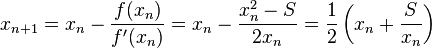For example, using this method the following script takes about 10 iterations to compute the square root of 900 with an error less than 1e-6.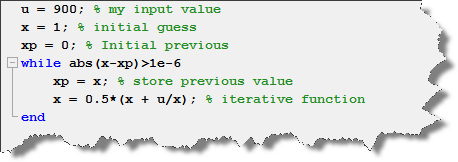Typically, in Simulink, blocks are executed once per time step (it is not always true, but let's assume that for today). For example, if I implement the equations above at the top level of a model with a fixed step solver and a sample time of 1 second, it will take ten seconds of simulation before the algorithm converges: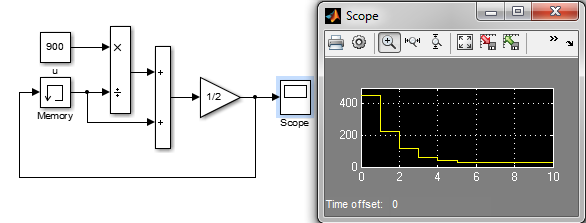As you can see, for a constant input, the algorithm slowly converges. But what if the input changes constantly? And what about the first steps where the answer is wrong?

We clearly need the algorithm to iterate multiple times at the same time step. To do that, you need an Iterator Subsystem: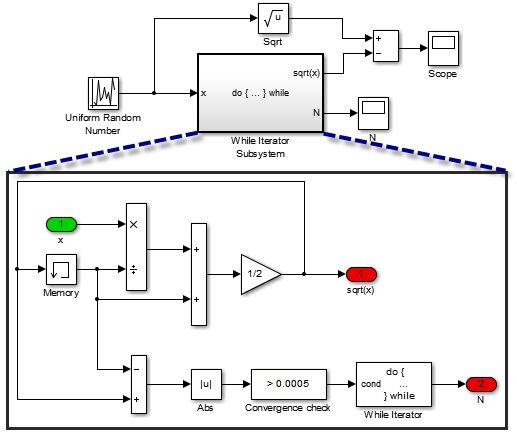With this implementation, at every step you will get an answer within the specified tolerance.

Starting with results for the previous step

In the implementation above, if we start the search with an initial guess of 1 every step, this is not very efficient. To help with that, you can start the search with the result of the previous time step by configuring the While Iterator Subsystem to hold its state: